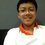# Prime factoring, remove multiple signs and find a prime!

We all know that prime factoring is to factorize a number to multiplications of primes, for example, $4=2 \times 2$. (Note: arrange the primes from small to big.) Remove the multiple signs, you'll get a number: 22. Repeat the steps, $22= 2 \times 11$. Remove the multiple signs to get 211, which is a prime. Now, lets do this for 6, which you can find a prime in just one step: $6=2 \times 3$, get 23, a prime. 8 is a bit tricky, you'll need to do a number of times to get an 18 or 19 digit number (I lost my draft paper). How about the other numbers?Note by Takeda Shigenori
6 years, 8 months ago

This discussion board is a place to discuss our Daily Challenges and the math and science related to those challenges. Explanations are more than just a solution — they should explain the steps and thinking strategies that you used to obtain the solution. Comments should further the discussion of math and science.

When posting on Brilliant:

• Use the emojis to react to an explanation, whether you're congratulating a job well done , or just really confused .
• Ask specific questions about the challenge or the steps in somebody's explanation. Well-posed questions can add a lot to the discussion, but posting "I don't understand!" doesn't help anyone.
• Try to contribute something new to the discussion, whether it is an extension, generalization or other idea related to the challenge.

MarkdownAppears as
*italics* or _italics_ italics
**bold** or __bold__ bold
- bulleted- list
• bulleted
• list
1. numbered2. list
1. numbered
2. list
Note: you must add a full line of space before and after lists for them to show up correctly
paragraph 1paragraph 2

paragraph 1

paragraph 2

[example link](https://brilliant.org)example link
> This is a quote
This is a quote
    # I indented these lines
# 4 spaces, and now they show
# up as a code block.

print "hello world"
# I indented these lines
# 4 spaces, and now they show
# up as a code block.

print "hello world"
MathAppears as
Remember to wrap math in $$ ... $$ or $ ... $ to ensure proper formatting.
2 \times 3 $2 \times 3$
2^{34} $2^{34}$
a_{i-1} $a_{i-1}$
\frac{2}{3} $\frac{2}{3}$
\sqrt{2} $\sqrt{2}$
\sum_{i=1}^3 $\sum_{i=1}^3$
\sin \theta $\sin \theta$
\boxed{123} $\boxed{123}$

Sort by:

The number is 311 for 9.

- 6 years, 8 months ago

I got an idea. I guess this is where programming is going to help us. Maybe I can try making a program which will factorize the number and then add the values. If I get any answers then I'll tell you. If anyone of you have any idea about how it would be then plz reply.

Also (of-course) there are 2 exceptions, which are naturally 0 and 1.

- 6 years, 2 months ago

I don't think you can even prove that there are infinitely many composite numbers that can be reduced to a prime... Also, I don't think you can prove there are infinitely many numbers that can't be reduced to a prime... This looks like an operation that, no matter what question you ask, it will probably be an open one, and very difficult to solve. Unless I'm missing some trivial solution here, but I doubt it.

- 6 years, 4 months ago

In the case of 12=2x3x3 and 233 is prime...

- 6 years, 2 months ago

That's crazy!

Can we prove that it's always possible to make a prime froma composite? I tried and it seems pretty complicated. It works for all the values iI tried though.

- 6 years, 2 months ago

Yea, that's what I'm thinking too. It has worked for every single case I've tried, but I can't seem to prove it. I've been trying to find a case where the successive numbers turn into a recursive loop, therefore disproving the conjecture of all composites turn prime, but no luck so far.

The tricky part is the concatenation, can't seem to find a mathematically rigorous way to define it.

- 6 years, 2 months ago

Yeah, it's hard to do it by just randomly looking for one.

What is the maximum number of steps to get to a prime?

Do numbers ever get smaller?

Will this produce every prime if we do every number? Which specific numbers will it not produce?

- 6 years, 2 months ago

Be aware! Maybe this will become Takeda's Conjecture and it may be remain unsolve for centuries! Haha just kidding...

- 6 years, 2 months ago

Takeda's conjecture :) But I don't think this conjecture will actually be proved as there seems to be no way to define the concatenation part

- 6 years, 2 months ago

64 = 2 x 2 x 2 x 2 x 2 x 2 = 222222

now 222222 = 2 × 3 × 7 × 11 × 13 × 37

= 237111337

237111337 = 29 × 101 × 80953 = 2910180953

2910180953 = 853 × 3411701 = 8533411701

8533411701 = 3 x 181 x 367 x 42821 = 318136742821

318136742821 = 127 x 2505013723 = 1272505013723

1272505013723 is prime. Awesome. So what's the logic?

- 6 years, 2 months ago

8=3331113965338635107

- 6 years, 2 months ago

I dont understand...what is the purpose of doing this??

- 6 years, 2 months ago

3 = $1 \times 3$, get 13. That's very interesting!

- 6 years, 7 months ago

actually, $3 = 3$ and that's the end of it. The prime factorization doesn't include 1's, in that case you could put as many 1's as you like.

- 6 years, 4 months ago

If 1 could be included in a prime factorization then $3 = 1 \times 3 , 3 = 1 \times 1 \times 3 , ......$ so 1 cannot be included in a prime factorization ( Also 1 is not a prime )

- 6 years, 2 months ago